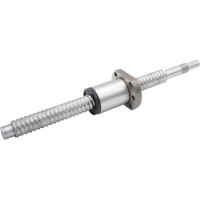18854719895

# 滚珠丝杠调整尺寸需四步

1.确定轴向载荷

Fb = 最大压缩（屈曲）载荷 (N)

fb = 基于端部支撑轴承的系数

dr = 螺杆根部直径 (mm)

Lc = 无支撑螺钉长度 (mm)

2.检查临界速度

nc = 临界转速 (rpm)

fc = 基于端部支撑轴承的系数

dr = 螺杆根部直径 (mm)

Lc = 无支撑螺钉长度 (mm)3.计算PV值

PV值

P = 螺钉和螺母之间的压力 (MPa)

FA = 螺母上的轴向载荷 (N)

A = 接触面积 (m2)

PV值

V= 丝杠和螺母之间的线速度 (m/s)

lhr = 螺杆每转的螺旋长度 (m)

rpm = 所需螺杆转速

4.考虑回车

Tb = 反向驱动扭矩 (Nm)

F = 轴向载荷（N)

P = 丝杠导程 (m)

η2 = 反向效率*

###### 相关新闻

•